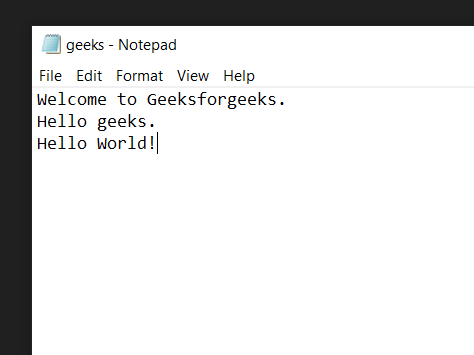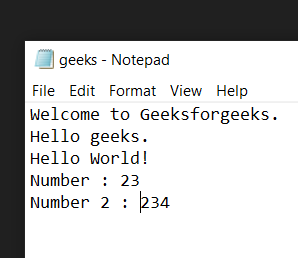GeeksforGeeks App
Open AppBrowser
Continue

# Python Program to Count Words in Text File

In this article, we are going to see how to count words in Text Files using Python.

### Example 1: Count String Words

First, we create a text file of which we want to count the number of words. Let this file be SampleFile.txt with the following contents:

#### File for demonstration:## Python3

 `# creating variable to store the``# number of words``number_of_words ``=` `0` `# Opening our text file in read only``# mode using the open() function``with ``open``(r``'SampleFile.txt'``,``'r'``) as ``file``:` `    ``# Reading the content of the file``    ``# using the read() function and storing``    ``# them in a new variable``    ``data ``=` `file``.read()` `    ``# Splitting the data into separate lines``    ``# using the split() function``    ``lines ``=` `data.split()` `    ``# Adding the length of the``    ``# lines in our number_of_words``    ``# variable``    ``number_of_words ``+``=` `len``(lines)`  `# Printing total number of words``print``(number_of_words)`

Output:

`7`

Explanation:

• Creating a new variable to store the total number of words in the text file. And then open the text file in read-only mode using the open() function.
• Read the content of the file using the read() function and storing them in a new variable. And then split the data stored in the data variable into separate lines using the split() function and then storing them in a new variable. And add the length of the lines in our number_of_words variable.

### Example 2: Count the number of words, not Integer

File for demonstration:Below is the implementation:

## Python3

 `# creating variable to store the``# number of words``number_of_words ``=` `0` `# Opening our text file in read only``# mode using the open() function``with ``open``(r``'SampleFile.txt'``,``'r'``) as ``file``:` `    ``# Reading the content of the file``    ``# using the read() function and storing``    ``# them in a new variable``    ``data ``=` `file``.read()` `    ``# Splitting the data into separate lines``    ``# using the split() function``    ``lines ``=` `data.split()` `    ``# Iterating over every word in``    ``# lines``    ``for` `word ``in` `lines:` `        ``# checking if the word is numeric or not``        ``if` `not` `word.isnumeric():         ` `            ``# Adding the length of the``            ``# lines in our number_of_words``            ``# variable``            ``number_of_words ``+``=` `1` `# Printing total number of words``print``(number_of_words)`

Output:

`11`

Explanation: Create a new variable to store the total number of words in the text file and then open the text file in read-only mode using the open() function. Read the content of the file using the read() function and storing them in a new variable and then split the data stored in the data variable into separate lines using the split() function and then storing them in a new variable, Iterating over every word in lines using the for loop and check if the word is numeric or not using the isnumeric() function then add 1 in our number_of_words variable.

My Personal Notes arrow_drop_up